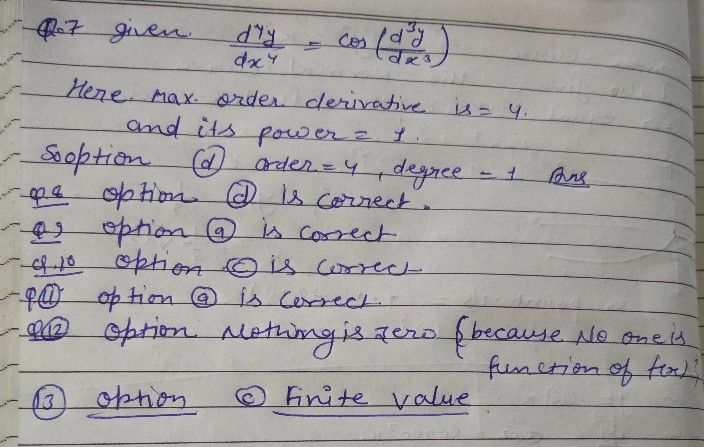Symbol
Problem$7.The$ order and degree of the $ODE\dfrac {a^{4}y} {dx^{4}}=cos\left(\dfrac {a^{3}y} {dx^{3}}$ is (A) $Ordet=4,$ degree = $1ndefineC$ (B) $Order=3$ degree = 1 (C) $Ordcr=3,$ degree $=defined$ (D) $Order=4,$ degree = 1 8. What are Fourier coefficients? a) The terms which consist of the fourier series along with their sine $orcosine$ values. b) The terms that are obtained through fourier series. c) The terms that are present in a fourier series d) The terms which are of resemblance to fourier transform in a fourier series are called fourier series coefficients 9. What is the disadvantage of exponential Fourier series? a) It cannot be easily visualized as sinusoids b) It is not easily visualized c) It is tough to calculate d) It is hard for manipulation 10. How does Fourier series make it easier to represent periodic signals? a) Harmonically related b) Periodically related c) Sinusoidally related d) Exponentially related 11. A "periodic function" is given by a function which a) $saiisfiesf\left(t+T\right)=f\left(t\right)$ b) $Satisies$ $f\left(t-T\right)=f\left(t\right)$ c) $satisf1es$ $f\left(t+T\right)=-f\left(t\right)$ d) $saiisf1esf\left(t-T\right)=-f\left(t\right)$ 12. If the function f(x) is even, then which of the following is $zero7$ a) bn $b\right)a_{n}$ c) ao d) nothing is zero 13. The b, coefficient for the $f\left(x\right)=x^{2}is$ a) zero b) infinite value c) finite value d) can't be found
Calculus
Search count: 106
SolutionQanda teacher - yogi123